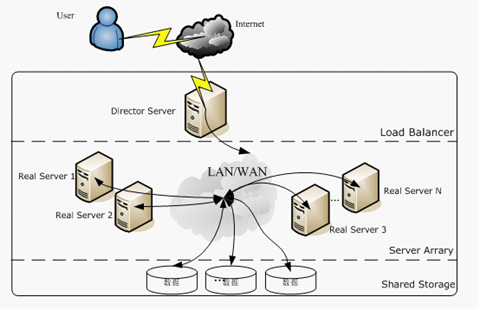深入理解Lucene默认打分算法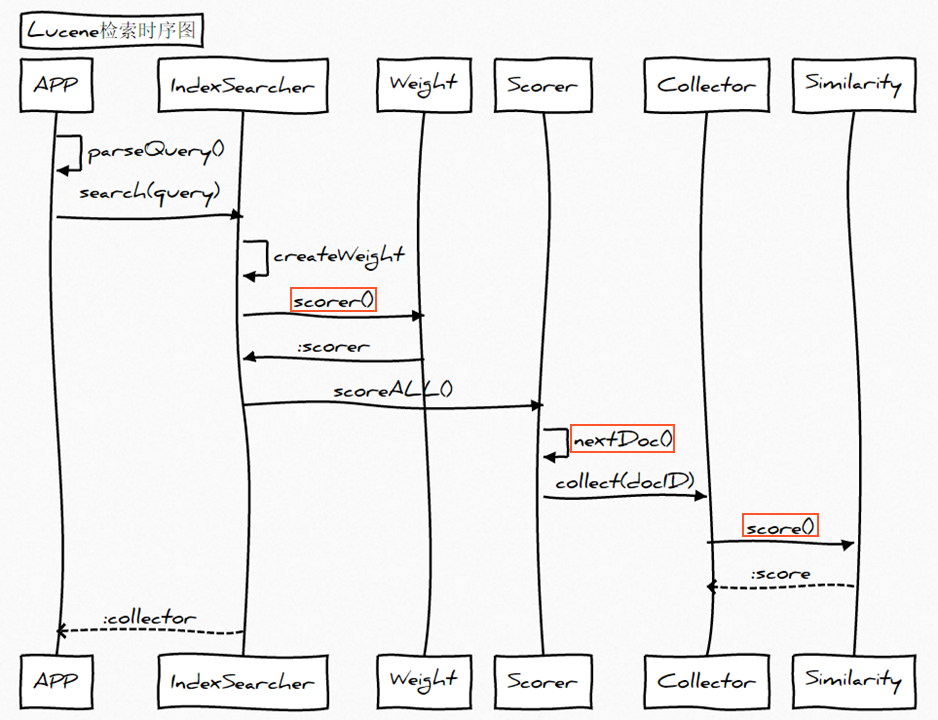匹配文档的打分因子

• 文档权重(Document boost)：在索引时给某个文档设置的权重值。
• 域权重(Field boost)：在查询的时候给某个域设置的权重值。
• 调整因子(Coord)：基于文档中包含查询关键词个数计算出来的调整因子。一般而言，如果一个文档中相比其它的文档出现了更多的查询关键词，那么其值越大。
• 逆文档频率(Inerse document frequency)：基于Term的一个因子，存在的意义是告诉打分公式一个词的稀有程度。其值越低，词越稀有(这里的值是指单纯的频率，即多少个文档中出现了该词；而非指Lucene中idf的计算公式)。打分公式利用这个因子提升包含稀有词文档的权重。
• 长度归一化(Length norm)：基于域的一个归一化因子。其值由给定域中Term的个数决定(在索引文档的时候已经计算出来了，并且存储到了索引中)。域越的文本越长，因子的权重越低。这表明Lucene打分公式偏向于域包含Term少的文档。
• 词频(Term frequency)：基于Term的一个因子。用来描述给定Term在一个文档中出现的次数，词频越大，文档的得分越大。
• 查询归一化因子(Query norm)：基于查询语句的归一化因子。其值为查询语句中每一个查询词权重的平方和。查询归一化因子使得比较不同查询语句的得分变得可行，当然比较不同查询语句得分并不总是那么易于实现和可行的。

Lucene的打分公式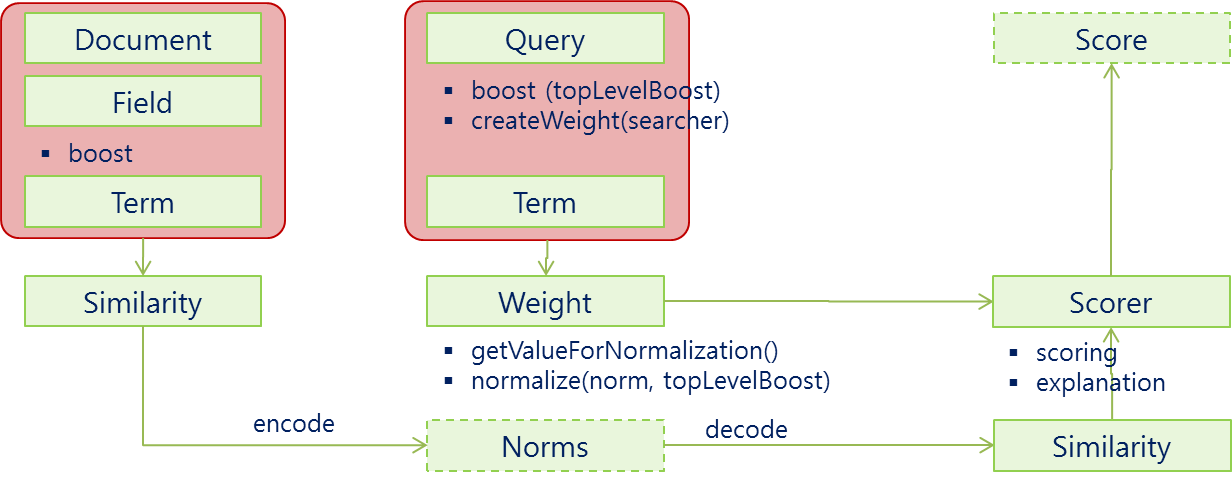Lucene概念上的打分公式是这样的：（TF/IDF公式的概念版）

$$score(q,d)=coord(q,d) * queryBoost(q) * \frac{V(q) * V(d)}{|V(q)|} * lengthNorm(d) * docBoost(d)$$

$$score(q,d) = coord(q,d) * queryBoost(q) * \sum_{t \in q}(tf(t \in q) * idf(t)^2 * boost(t) * norm(t,d))$$

• 匹配到的关键词越稀有，文档的得分就越高。
• 文档的域越小(包含比较少的Term)，文档的得分就越高。
• 设置的权重(索引和搜索时设置的都可以)越大，文档得分越高。

coord(q,d)

public float coord(int overlap, int maxOverlap) {
return overlap / (float)maxOverlap;
}

• overlap：检索命中query中term的个数
• maxOverlap：query中总共的term个数

queryNorm(q)

queryNorm(q)是查询权重对得分的影响，这个因素对所有文档都是一样的值，所以它不影响排序结果。比如如果我们希望所有文档的评分大一点，那么我们就需要设置这个值。它的计算公式如下：

public float queryNorm(float sumOfSquaredWeights) {
return (float)(1.0 / Math.sqrt(sumOfSquaredWeights));
}

tf(t in d)

public float tf(float freq) {
return (float)Math.sqrt(freq);
}

idf(t)

public float idf(long docFreq, long numDocs) {
return (float)(Math.log(numDocs/(double)(docFreq+1)) + 1.0);
}

• docFreq 指的是项出现的文档数，就是有多少个文档符合这个搜索
• numDocs 指的是索引中有多少个文档。

• this book is about english
• this book is about chinese
• this book is about japan

boost(t)

norm(t,d)

• chinese
• chinese book

m(t,d) = doc.getBoost()· lengthNorm· ∏ f.getBoost()

public float lengthNorm(FieldInvertState state) {
final int numTerms;
if (discountOverlaps)
numTerms = state.getLength() - state.getNumOverlap();
else
numTerms = state.getLength();
return state.getBoost() * ((float) (1.0 / Math.sqrt(numTerms)));
}

• TF-IDF 算法是以 term为基础的，term就是最小的分词单元，这说明分词算法对基于统计的ranking无比重要，如果你对中文用单字切分，那么就会损失所有的语义相关性，这个时候搜索只是当做一种高效的全文匹配方法。
• 按照规则1 某个词或短语在一篇文章中出现的次数越多，越相关。一定要去除掉stop word，因为这些词出现的频率太高了，也就是TF的值很大，会严重干扰算分结果。
• TF在生成索引的时候，就会计算出来并保存，而IDF是在query的时候获取，包含t的文档数= length（term的posting list)。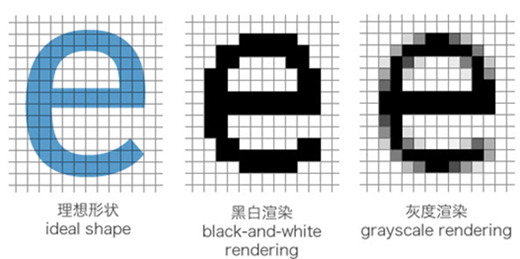网站与APP开发中的字体设置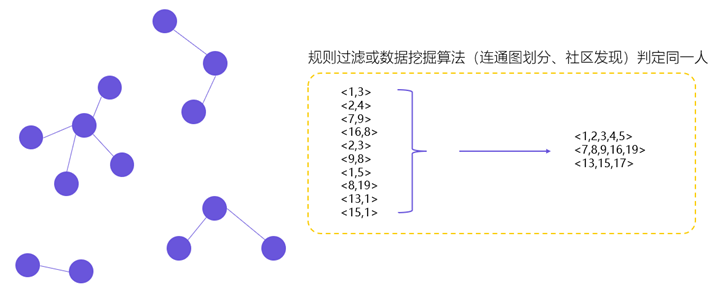用户体系搭建之ID-Mapping